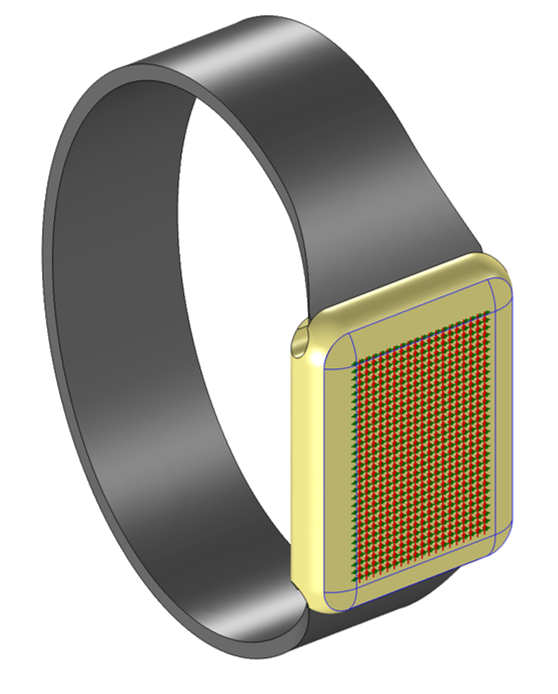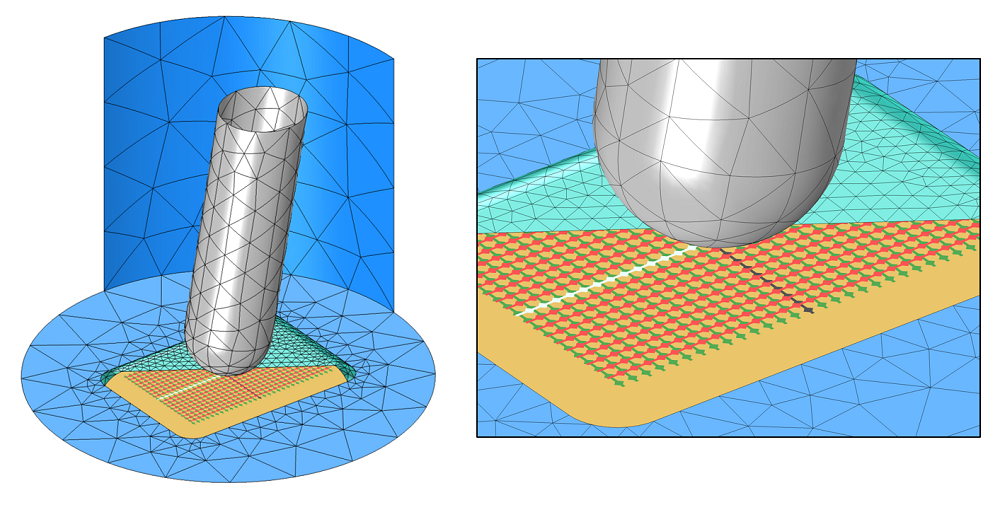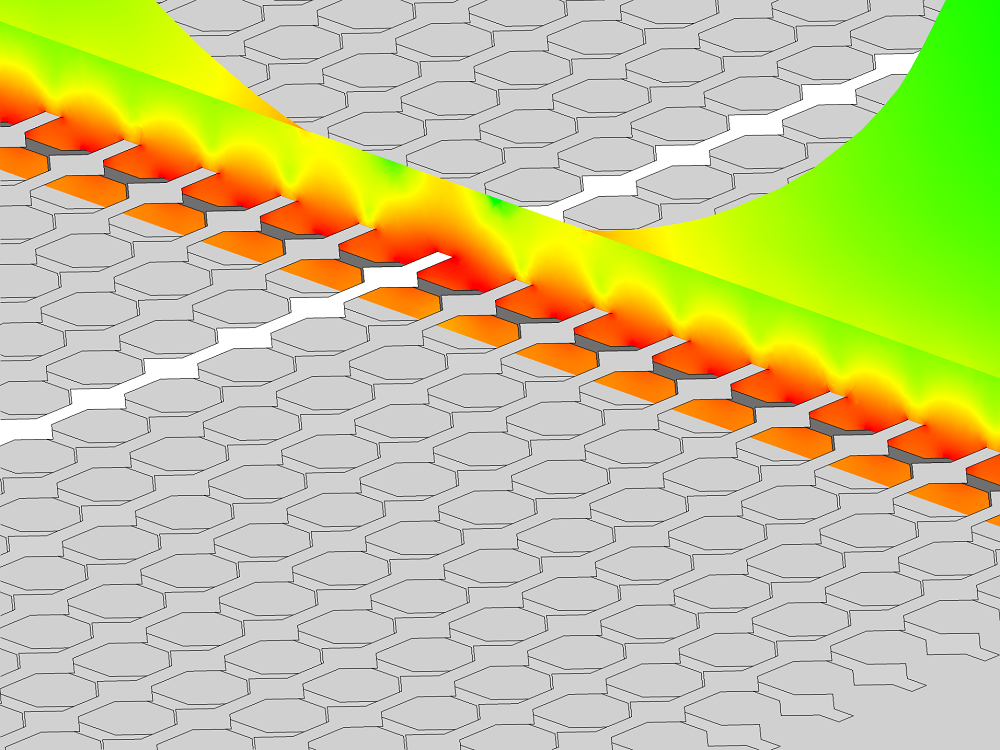# 消费电子产品中的电容触摸屏仿真分析

2015年 1月 1日

### 电容传感简介### 模拟腕表中的电容传感器#### 所使用的边界条件#### 所用材料### 使用自适应网格细化所获得的精确解

0.125 （缺省“标准”网格设定） 1.7 10 28%
0.6 （第一次自适应网格细化后） 2.2 20 6%
2.3 （第二次细化） 4.8 84 2%
7.7 （第三次细化） 14 711 0.6%
24.4 （第四次细化） 47 2,960 N/A

### 计算电容矩阵

\left\{ \begin{array}{c} Q_1\\ \vdots \\Q_n \end{array} \right\} = \left[ \begin{array}{ccc} C_{11} & \ldots & C_{1n} \\ \vdots & \ddots & \vdots \\ C_{n1} & \ldots & C_{nn} \\ \end{array} \right] \left\{ \begin{array}{c} V_1\\ \vdots \\V_n \end{array} \right\}

C_{ii}=\frac{2}{V_i^2} \int \limits_\Omega W_e d\Omega

V_k = \begin{cases} \begin{array}{c c} 0 & j \neq i \\ V_i & j = i\end{array} \end{cases}

C_{ij}=\frac{2}{V_i V_j} \int \limits_\Omega W_e d\Omega-\frac{1}{2}\left( \frac{V_i}{V_j}C_{ii} + \frac{V_j}{V_i}C_{jj}\right)

V_k = \begin{cases} \begin{array}{c c} 0 &k\neq i,j\\ V_i & k=i \\V_j & k=j \end{array} \end{cases}